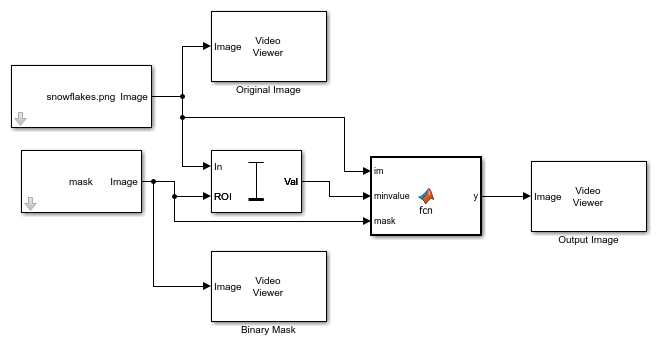# Find Minimum Value in ROI

This example shows how to calculate the minimum value in an image ROI by using the `2-D` `Minimum` block. By using the minimum value, the model removes indistinct pixels in the image regions.

### Example Model

The model reads the original image and the binary mask comprising the ROI for which the minimum value has to be computed. The original image consists of regions with large image structures that are circular in shape and regions with small, indistinct image structures. The binary mask isolates the indistinct structures from the distinct image structures.

Load the binary mask containing the ROI to MATLAB workspace. The ROI corresponding to indistinct structures have intensity value 1 in the binary mask.

```load('binarymask.mat'); ```

Open the model.

```modelname='ex_blk2dminimum'; open_system(modelname); ```For the `2-D` `Minimum` block to output only the computed minimum, the `Mode` parameter of the block is set to `Value`. To perform ROI processing, the `Find` `the` `minimum` `value` `over` parameter is set to `Entire` `input`. The ROI input to the `2-D` `Minimum` block is a binary image. Hence, the `ROI` `Type` parameter is set to `Binary` `mask`. The block computes the minimum value of the pixels in the original image that lie in the ROI specified by the binary mask.

The MATLAB `function` `block` replaces pixel values in the ROI with the computed minimum.

### Simulate and Display Results

The model outputs a clean image with only distinct image structures.

``` sim(modelname); ```